• +91 9971497814
• info@interviewmaterial.com

# RD Chapter 15- Areas of Parallelograms and Triangles Ex-15.2 Interview Questions Answers

### Related Subjects

Question 1 : If the given figure, ABCD is aparallelogram, AE  DC and CF  AD. If AB =16 cm. AE =8 cm and CF =10 cm, find AD.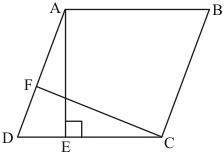Given: Here in the question it is given
(1) ABCD is a parallelogram,
(2)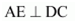and
(3)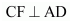, AB = 16 cm
(4) AE = 8cm
(5) CF = 10cm
Calculation: We know that formula for calculating theTherefore,
Area of paralleogram ABCD = DC × AE (Taking base as DC and Height as AE )
Area of paralleogram ABCD = AB × AE (AB = DC as opposite side of the parallelogram are equal)
Therefore,
Area of paralleogram ABCD = 16 × 8 ……(1)
Taking the base of Parallelogram ABCD as AD we get
Area of paralleogram ABCD = AD × CF (taking base as AD and height as CF)
Area of paralleogram ABCD = AD × 10 ……(2)
Since equation 1 and 2 both represent the Area of the same Parallelogram ABCD , both should be equal.
Hence fro equation (1) and (2),
This means that,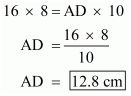Hence we get the result as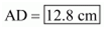Question 2 : In Q.No. 1, if AD = 6 cm, CF = 10 cm, and AE = 8 cm, find AB.

Given: Here in the question it is given that
(1) ABCD is a parallelogram,
(2)and
(3)(5) AE = 8cm
(6) CF = 10cm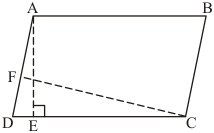To Find : AB =?
Calculation: We know that formula for calculating the
To Find : AB =?
Calculation: We know that formula for calculating the
Area of paralleogram = base × height
Therefore,
Area of paralleogram ABCD = DC × AE (Taking base as DC and Height as AE )
Area of paralleogram ABCD = AB × AE (AB = DC as opposite side of the parallelogram are equal)
Therefore, Area of paralleogram ABCd = 16 × 8
Area of Parallelogram ABCD = AB× 8 ……(1)
Taking the base of Parallelogram ABCD as AD we get
Area of paralleogram ABCD = AD × CF (taking base as AD and height as CF)
Area of paralleogram ABCD = 6 × 10 ……(2)
Since equation 1and 2 both represent the Area of the same Parallelogram ABCD , both should be equal.
Hence equation 1 is equal to equation 2
Which means that,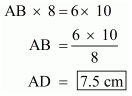Hence we got the measure of AB equal to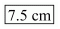Question 3 : Let ABCD be a parallelogram of area 124 cm2. If E and F are the mid-points of sides AB and CD respectively, then find the area of parallelogram AEFD.

Given: Here in the question it is given that
(1) Area of paralleogram ABCD = 124 cm2
(2) E is the midpoint of AB, which means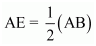(3) F is the midpoint of CD, which means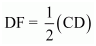To Find : Area of Parallelogram AEFD
Calculation: We know that formula for calculating the
Area of Parallelogram = base × height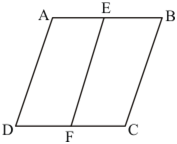Therefore,
Area of paralleogram ABCD = AB × AD (Taking base as AB and Height as AD ) ……(1)
Therefore,
Area of paralleogram AEFD = AE × AD (Taking base as AB and Height as AD ) ……(2)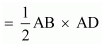(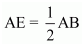)
=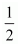Area of Parallelogram ABCD (from equation1)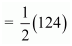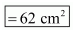Hence we got the result Area of Parallelogram AEFDQuestion 4 :
If ABCD is a parallelogram, then prove that
ar (ΔABD) = ar (ΔBCD) = ar (ΔABC) = ar (ΔACD) = 1212 ar (||gm ABCD)

Given: Here in the question it is given that
(1) ABCD is a Parallelogram
To Prove :
(1)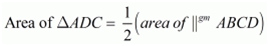(2)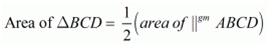(3)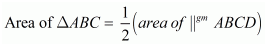(4)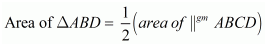Construction: Draw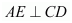Calculation: We know that formula for calculating the
Area of Parallelogram = base × height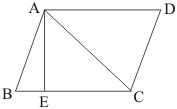Area of paralleogram ABCD = BC × AE (Taking base as BC and Height as AE ……(1)
We know that formula for calculating the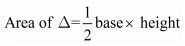Area of ΔADC =Base × Height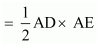(AD is the base of ΔADC and AE is the height of ΔADC)
=Area of Parallelogram ABCD (from equation1)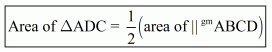Hence we get the resultSimilarly we can show that
(2)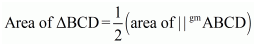(3)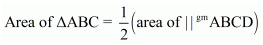(4)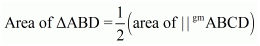Todays Deals### RD Chapter 15- Areas of Parallelograms and Triangles Ex-15.2 Contributorskrishan

Name:
Email:

# Latest News# 9000 interview questions in different categories